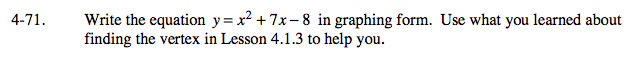### Home > A2C > Chapter 4 > Lesson 4.2.1 > Problem4-71

4-71.

Write the equation y = x2 + 7x − 8 in graphing form. Use what you learned about finding the vertex in Lesson 4.1.3 to help you. Homework Help ✎Complete the square. See the online help for problem A2C 4-40 part (a) if you need help.

The equation is y = (x + 3.5)2 − 20.25.